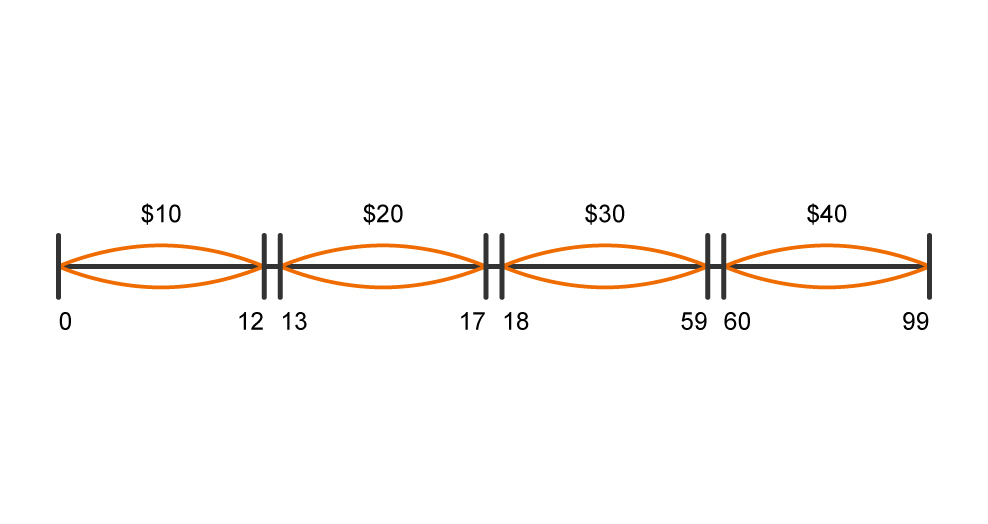# Test Design TechniquesMany people test and write test cases, but not many use special test design techniques. Gradually, gaining experience, they realize that they constantly do the same work, which is subject to specific rules. And then they find that all these rules have already been described.

We suggest you familiarize yourself with a brief description of the most common test design techniques:

• Equivalence Partitioning (EP). The equivalent class includes a certain number of some values that are supposed to be processed and output the same result for all representatives of this equivalent class. As an example, you have a range of valid values from 1 to 10, you must choose one correct value within the interval, say 5, and one wrong value outside the interval – 0.

To make it easier to understand, let’s assume that there is a simple script or program that invites us to enter a person’s age in a text field with a capacity of 2 characters, and below there is a button for sending data. The result of this program should be the cost of medical insurance, depending on the indicator that we enter. In accordance with the functional specification, all ages were divided into four categories. The first is Children (includes 0 to 12 years), The second category is Juveniles (includes 13 to 17 years), The third category is Adults (includes 18 to 59 years), and the last fifth category is the Elderly (includes 60 to 99 years old). Depending on the category to which the person belongs, the cost of medical insurance will be calculated:

• Children – 10 \$ a month
• Juveniles – \$ 20 per month
• Adults – 30 \$ per month
• Elderly – 40 \$ per month

In this case, all input data can be divided into 4 equivalent classes. As shown in the figure below.Question: How many test cases do we need to test all equivalent classes and which ones?

To test all four equivalent classes, we will need to run tests with one representative from each Equivalent class.

Why can not we check for example 0,1,2,3 … and so on, sorting through all the options that the user can enter? It’s simple, because we do extra work. Cover all the equivalent classes can be only four tests.

In the first case, take any number from 0 to 12 (for example, 5)

In the second case, take any number from 13 to 17 (for example, 15)

In the third case, we take any number from 18 to 59 (for example, 36)

In the fourth case, take any number from 60 to 99 (for example, 78)

In total, we covered all four equivalent classes with four tests.

For a more accurate check, you also need to check the boundary values, since bugs often appear on the boundaries of equivalent classes.

• Bojundars Value Analasis (BVA). If we take the example above, as the values for positive testing, we choose the minimum and maximum boundaries (1 and 10), and the values are larger and smaller than the boundaries (0 and 11). Analysis Boundary values can be applied to fields, records, files, or to any kind of entities with constraints.
• Cause / Effect (CE). This is, as a rule, the input of combinations of conditions (causes), to obtain a response from the system (Effect). For example, you check the ability to add a client using a specific screen form. To do this, you will need to enter several fields, such as “Name”, “Address”, “Phone Number” and then click “Add” – this “Reason”. After clicking the “Add” button, the system adds the client to the database and displays its number on the screen – this is “Consequence”.
• Error Guessing (EG). This is when the test analyst uses his knowledge of the system and the ability to interpret the specification for “guessing” under what input conditions the system can produce an error. For example, the specification says: “the user must enter the code.” Analyst test, will think: “What if I do not enter the code?”, “What if I enter the wrong code?”, And so on. This is the error prediction.
• Exhaustive Testing (ET) is an extreme case. Within this technique, you should check all possible combinations of input values, and in principle, this should find all the problems. In practice, the application of this method is not possible, because of the huge number of input values.

Next time we will consider the practical application of test design techniques in the development of test cases.